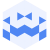Am 27. Oct. 2022 | 19:00 Uhr

# Oct 27 2022 | Tresor Linz I canceled but not canceled I Passepartout DuoCreated at 24. Oct. 2022

111 Ansichten
##### by Tresor Linz

<<<<<<<<<<<<<<<<<<<<<<<<<<<<<<<<<<<<<<<<<<<<<<<<<<<<<<<<<
Passepartout Duo
<<<<<<<<<<<<<<<<<<<<<<<<<<<<<<<<<<<<<<<<<<<<<<<<<<<<<<<<<

Live Set: Circo Pobre

The centerpiece of this live set is the Chromaplane, a new electronic instrument the Duo has created over the past year. With a pickup coil in each hand, the performers trace shapes above the instrument's flat surface to unveil its hidden landscape of oscillators buzzing in the electromagnetic field.
The four parts are defined by taking both a temporal and spatial approach to the sound and create the arch of a listening journey.

Passepartout Duo

Drawing from a carefully selected palette of electro-acoustic textures and shapeshifting rhythms, Passepartout Duo’s work investigates the way in which we listen to and connect with sound. Continually reassessing the tools they use to create their music, the group is continually developing a specialized and evolving ecosystem of handmade musical instruments that have ranged from analog electronic circuits and conventional percussion, to room-size textile installations and found objects.

Looking for ways of sustaining an independent musical career and shaping an international network of artists and musicians, the group has been on a nearly continuous world tour ever since they began collaborating in 2015, and their experiences traveling have heavily influenced their unconventional creative process.

Passepartout Duo has been the recipient of dozens of artist residency opportunities around the world including The Watermill Center (US), the Swatch Art Peace Hotel (CN), the Rogers Art Loft (US), and the Embassy of Foreign Artists (CH). Their multidisciplinary approach has also led them to exhibit both their own creations and their collaborations with fellow artists at venues such as Casino Luxembourg (LU), the Nakanojo Biennale (JP), Aarhus Artspace (DK), and Fanrong Museum (CN).

The duo was selected for SHAPE+ Platform’s roster of artists 2022-2023.

Passepartout Duo is formed by pianist Nicoletta Favari (IT) and percussionist Christopher Salvito (US/IT).

<<<<<<<<<<<<<<<<<<<<<<<<<<<<<<<<<<<<<<<<<<<<<<<<<<<<<<<<<
Tresor Linz
<<<<<<<<<<<<<<<<<<<<<<<<<<<<<<<<<<<<<<<<<<<<<<<<<<<<<<<<<

Raum für Klangkunst und künstlerische Experimente.

Space for sound art and artistic experiments.

##### Share & Embed
Embed this Video